Courses

# Test: Intro Differential Equations

## 10 Questions MCQ Test Mathematics (Maths) Class 12 | Test: Intro Differential Equations

Description
This mock test of Test: Intro Differential Equations for JEE helps you for every JEE entrance exam. This contains 10 Multiple Choice Questions for JEE Test: Intro Differential Equations (mcq) to study with solutions a complete question bank. The solved questions answers in this Test: Intro Differential Equations quiz give you a good mix of easy questions and tough questions. JEE students definitely take this Test: Intro Differential Equations exercise for a better result in the exam. You can find other Test: Intro Differential Equations extra questions, long questions & short questions for JEE on EduRev as well by searching above.
QUESTION: 1

### The dif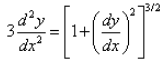is a:

Solution:

We know to calculate degree or order, the powers of derivatives should be integer.

on squaring both sides, we get a differential equation with integers as the power of derivatives

so order is the highest derivative, which is 2

and degree is the power of highest degree which is again 2

QUESTION: 2

### The differential equation for the equation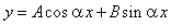is :

Solution:

y = Acos(αx) + Bsin(αx)

dy/dx = -Aαsin(αx) + Bαcos(αx)

d2y/dx2 = -Aα2cos(αx) - Bα2sin(αx)

= -α2(Acos(αx) + Bsin(αx))

= -α2 * y

d2y/dx2 + α2*y = 0

QUESTION: 3

### Formation of the differential equation of the family of curves represented by  y = Ae2x + Be-2x is :

Solution:

y = Ae2x + Be-2x
dy/dx = 2Ae2x – 2Be-2x
d2y/dx2 = 4Ae2x + 4Be-2x
= 4*y
d2y/dx2 – 4y = 0

QUESTION: 4

The degree of the differential equation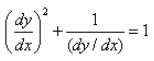Solution:

Given equation is :

(dy/dx)2 + 1/(dy/dx) = 1

((dy/dx)3 + 1)/(dy/dx) = 1

(dy/dx)3 +1 = dy/dx

So, final equation is

(dy/dx)3 - dy/dx + 1 = 0

So, degree = 3

QUESTION: 5

Differential equation representing the family of curves given by y = ax + x2 is:

Solution:

The answer is C. We eliminate constants.

We have

y=ax+x2

Differentiating with respect to x,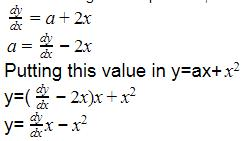QUESTION: 6

The order of the differential equation: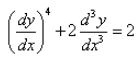Solution: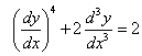Order of the D.E. is 3
Order of a differential equation is the order of the highest derivative present in the equation.

QUESTION: 7

Formation of the differential equation corresponding to the ellipse major axis 2a and minor axis 2b is:

Solution:

Equation of ellipse :

x2/a2 + y2/b2 = 1

Differentiation by x,

2x/a2 + (dy/dx)*(2y/b2) = 0

dy/dx = -(b2/a2)(x/y)

-(b2/a^2) = (dy/dx)*(y/x) ----- eqn 1

Again differentiating by x,

d2y/dx2 = -(b2/a2)*((y-x(dy/dx))/y2)

Substituting value of -b2/a2 from eqn 1

d2y/dx2 = (dy/dx)*(y/x)*((y-x(dy/dx))/y2)

d2y/dx2 = (dy/dx)*((y-x*(dy/dx))/xy)

(xy)*(d2y/dx2) = y*(dy/dx) - x*(dy/dx)2

(xy)*(d2y/dx2) + x*(dy/dx)2- y*(dy/dx) = 0

QUESTION: 8

The differential equation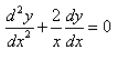is a solution of the equation:

Solution:

Solving second order differential equation with variable coefficients becomes a bit lengthy and complicated. So, its better to check by options.

On checking option A :

y = A/x + B

dy/dx = -A/x2

d2y/dx= (2A)/x3

So,

d2y/dx2 + (2/x)*(dy/dx) = 0

(2A)/x3 + (2/x)*((-A)/x2) = 0

(2A - 2A)/x3 = 0

0 = 0

LHS = RHS

QUESTION: 9

The differential equation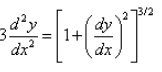is a:

Solution:

3*(d2y/dx2) = [1+(dy/dx)2]3/2
On squaring both side,
9*(d2y/dx2)2 = [1+(dy/dx)2]3
The order of the equation is 2. The power of the term determining the order determines the degree.
So, the degree is also 2.

QUESTION: 10

The order and degree of the differential equation: (y”)2 + (y”)3 + (y’)4 + y5 = 0 is:​

Solution:

The highest order derivative here is y’’. Therefore the order of the differential equation=2.
The highest power of the highest order derivative here is 3. Therefore the order of the differential equation=3.